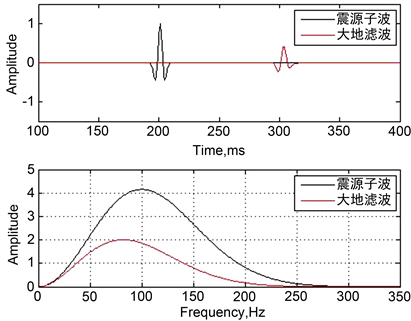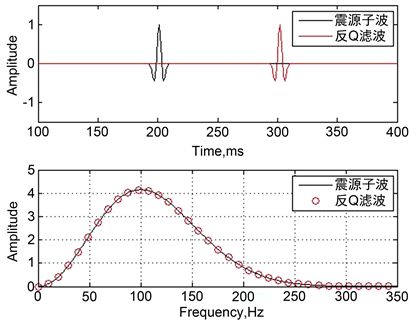﻿ 地震波吸收衰减模拟及反滤波处理

# 地震波吸收衰减模拟及反滤波处理Seismic Wave Absorption and Attenuation Simulation and Anti-Q Filter Processing

Abstract: The attenuation attribute of seismic wave in the stratum is expressed by the quality factor Q. The larger the Q value is, the weaker the attenuation is. The smaller the Q value is, the stronger the attenuation is. Anti-Q filtering is a technique to compensate the effect of geodetic filtering. Under the condition of known Q value, the quality of seismic data can be improved by using anti-Q filtering. In this paper, the attenuation effect of seismic wave is simulated numerically, and the anti-Q filtering is carried out to eliminate the attenuation effect of strata on seismic wave, and good results are obtained.

1. 概述

2. 方法原理

2.1. 品质因子Q

$\frac{1}{Q}=\frac{\Delta E}{2\pi E}$ (1)

2.2. 影响地震波衰减的因素

2.3. Futterman模型

Futterman对统一的黏弹性介质模型进行了研究，率先总结出了品质因子与频率的关系式，后人将其所做工作称为Futterman模型 。由于Futterman模型相对简单，所需参数容易估算，是常用的衰减模型。

$A\left(f\right)={A}_{0}\left(f\right)\mathrm{exp}\left(-\frac{\pi f}{Q}t\right)$ (2)

$AMP\left(f\right)=\mathrm{exp}\left(-\frac{\pi f}{Q}t\right)$ (3)

$\phi \left(f\right)=H\left\{\mathrm{ln}\left[AMP\left(f\right)\right]\right\}=H\left\{\mathrm{ln}\left[\mathrm{exp}\left(-\frac{\pi f}{Q}t\right)\right]\right\}=-\frac{\pi t}{Q}H\left(f\right)$ (4)

$\begin{array}{l}S\left(f\right)=AMP\left(f\right)\mathrm{exp}\left[i\phi \left(f\right)\right]=\mathrm{exp}\left(-\frac{\pi f}{Q}t\right)\mathrm{exp}\left[-\frac{i\pi f}{Q}H\left(f\right)\right]\\ ⇒S\left(f\right)=\mathrm{exp}\left\{-\frac{\pi t}{Q}\left[f+iH\left(f\right)\right]\right\}\end{array}$ (5)

Futterman模型说明地震波呈负指数衰减并且是最小相位的。衰减量与频率f、时间t以及品质因子Q有关。频率和时间与衰减量成正比，品质因子与衰减量呈反比，这就是普遍存在于大地滤波过程中的地震波场衰减规律。Futterman模型可以较准确地描述地震波在介质中的衰减性质且表达式较为简洁，本文所有的运算均基于Futterman模型进行实现。

2.4. 吸收衰减模拟

$S\left(f\right)=\mathrm{exp}\left[-\frac{\pi t}{Q}\left(f+iH\left(f\right)\right)\right]$ (6)

$x\left(t\right)=\frac{1}{2\pi }\underset{0}{\overset{\infty }{\int }}R\left(f\right)S\left(f\right)\mathrm{exp}\left(i\omega t\right)\text{d}\omega$ (7)

2.5. 反Q滤波

$S{\left(f\right)}^{-1}=\mathrm{exp}\left[\frac{\pi t}{Q}G\left(f\right)\right]$ (8)

3. 模拟资料处理

3.1. 大地滤波数值模拟

$R\left(f\right)=\frac{2}{\sqrt{\pi }}\frac{{f}^{2}}{{f}_{m}^{2}}\mathrm{exp}\left(-\frac{{f}^{2}}{{f}_{m}^{2}}\right)$ (9)

3.2. 反Q滤波处理Figure 1. Amplitude spectrum and waveform after amplitude and phase attenuation (Q = 40, t = 100 ms)Figure 2. Waveform and amplitude spectrum after anti-Q compensation (Q = 40, t = 100 ms)

4. 结论

NOTES

*通讯作者。

 O’Doherty, R.F. and Anstey, N.A. (1971) Reflections on Amplitudes. Geophysical Prospecting, 19, 430-458.
https://doi.org/10.1111/j.1365-2478.1971.tb00610.x

 Richards, P.G. and Menke, W. (1983). The Apparent Attenuation of a Scattering Medium. Bulletin of the Seismological Society of America, 73, 1005-1021.

 陈增保, 陈小宏, 李景叶, 王本锋, 马莎. 一种带限稳定的反Q滤波算法[J]. 石油地球物理勘探, 2014, 49(1): 68-75.

 付勋勋, 徐峰, 秦启荣, 李培, 邵晓州. 基于改进的广义S变换求取地层品质因子Q值[J]. 石油地球物理勘探, 2012(3): 457-461.

 高静怀, 杨森林. 利用零偏移VSP资料估计介质品质因子方法研究[J]. 地球物理学报, 2007, 50(4): 1198-1209.

 何惺华. 利用零偏VSP资料提高地面地震资料分辨率的方法及其应用研究[J]. 石油物探, 2011, 50(5): 487-492.

 李红星, 陶春辉, 赵烽帆, 周建平. 双相介质地震勘探纵向分辨率研究[J]. 地球物理学报, 2013(3): 995-1002.

 蔡文涛, 胡光义, 范廷恩, 王宗俊, 聂妍. 谱模拟法提取地层品质因子[J]. 地球物理学进展, 2017, 29(2): 642-649.

 刘建华, 胥颐, 郝天珧. 地震波衰减的物理机制研究[J]. 地球物理学进展, 2004, 19(1): 1-7.

 马昭军, 刘洋. 地震波衰减反演研究综述[J]. 地球物理学进展, 2005, 20(4): 1074-1082.

 裴江云, 何樵登. 基于Kjartansson模型的反Q滤波[J]. 地球物理学进展, 1994, 9(1): 90-97.

 Futterman, W.I. (1962) Dispersive Body Waves. Journa1 of Geophysical Research, 67, 5279-5291.
https://doi.org/10.1029/JZ067i013p05279

Top